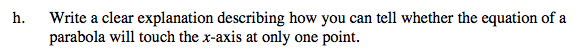### Home > A2C > Chapter 4 > Lesson 4.1.3 > Problem4-43

4-43.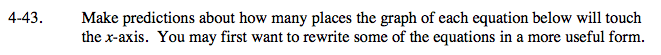How many x-intercepts will this equation have?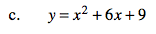Factor the right side in order to find the intercepts.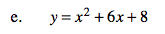Look at parts (a) through (d).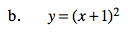See part (a).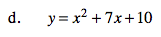Look at parts (a) through (c). Remember to factor first to find the x-intercepts.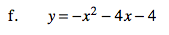Look at parts (a) through (d). Remember to factor −1 out.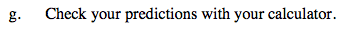What is true about all of the equations where the parabola touches the x-axis at only one point?